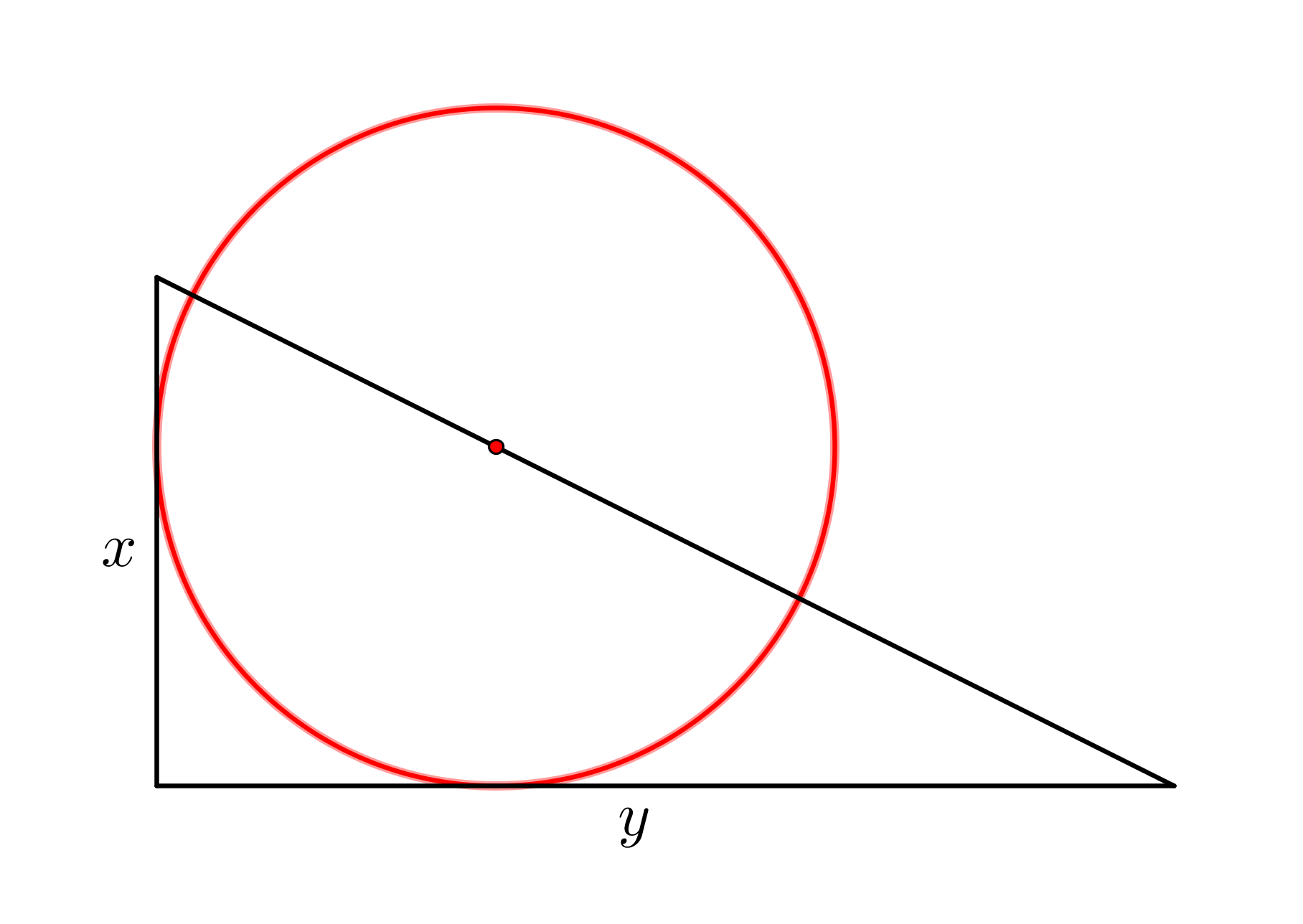#### You may also like### Fitting In

The largest square which fits into a circle is ABCD and EFGH is a square with G and H on the line CD and E and F on the circumference of the circle. Show that AB = 5EF. Similarly the largest equilateral triangle which fits into a circle is LMN and PQR is an equilateral triangle with P and Q on the line LM and R on the circumference of the circle. Show that LM = 3PQ### Look Before You Leap

Can you spot a cunning way to work out the missing length?### Triangle Midpoints

You are only given the three midpoints of the sides of a triangle. How can you construct the original triangle?

# Sitting Pretty

##### Age 14 to 16 Challenge Level:

A circle 'sits' on a right-angled triangle, touches two sides of the triangle and has its centre on the hypotenuse of the triangle. The circle has radius $r$ and the triangle has sides of length $x$ and $y$.Show that $\dfrac{1}{r} = \dfrac{1}{x} + \dfrac{1}{y}$.